Types of Matrices - Matrices and Determinants, Business Mathematics & Statistics

# Types of Matrices - Matrices and Determinants, Business Mathematics & Statistics Notes | Study Business Mathematics and Statistics - B Com

## Document Description: Types of Matrices - Matrices and Determinants, Business Mathematics & Statistics for B Com 2022 is part of Business Mathematics and Statistics preparation. The notes and questions for Types of Matrices - Matrices and Determinants, Business Mathematics & Statistics have been prepared according to the B Com exam syllabus. Information about Types of Matrices - Matrices and Determinants, Business Mathematics & Statistics covers topics like and Types of Matrices - Matrices and Determinants, Business Mathematics & Statistics Example, for B Com 2022 Exam. Find important definitions, questions, notes, meanings, examples, exercises and tests below for Types of Matrices - Matrices and Determinants, Business Mathematics & Statistics.

Introduction of Types of Matrices - Matrices and Determinants, Business Mathematics & Statistics in English is available as part of our Business Mathematics and Statistics for B Com & Types of Matrices - Matrices and Determinants, Business Mathematics & Statistics in Hindi for Business Mathematics and Statistics course. Download more important topics related with notes, lectures and mock test series for B Com Exam by signing up for free. B Com: Types of Matrices - Matrices and Determinants, Business Mathematics & Statistics Notes | Study Business Mathematics and Statistics - B Com
 1 Crore+ students have signed up on EduRev. Have you?

## Types of Matrices

This lesson describes a few of the more important types of matrices: transpose matrices, vectors, and different kinds of square matrices.

## Transpose Matrix

The transpose of one matrix is another matrix that is obtained by using rows from the first matrix as columns in the second matrix.

For example, it is easy to see that the transpose of matrix A is A'. Row 1 of matrix A becomes column 1 of A'; row 2 of A becomes column 2 of A'; and row 3 of A becomes column 3 of A'.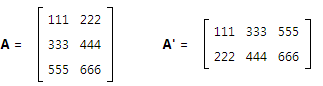Note that the order of a matrix is reversed after it has been transposed. Matrix A is a 3 x 2 matrix, but matrix A' is a 2 x 3 matrix.

With respect to notation, this website uses a prime to indicate a transpose. Thus, the transpose of matrix B would be written as B'.

## Vectors

Vectors are a type of matrix having only one column or one row.

Vectors come in two flavors: column vectors and row vectors. For example, matrix a is a column vector, and matrix a' is a row vector.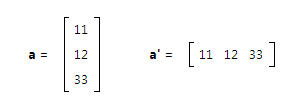We use lower-case, boldface letters to represent column vectors. And since the transpose of a column vector is a row vector, we use lower-case, boldface letters plus a prime to represent row vectors. Thus, vector b would be a column vector, and vector b' would be a row vector.

## Square Matrices

square matrix is an nn matrix; that is, a matrix with the same number of rows as columns. In this section, we describe several special kinds of square matrix.

• Symmetric matrix. If the transpose of a matrix is equal to itself, that matrix is said to be symmetric. Two examples of symmetric matrices appear below.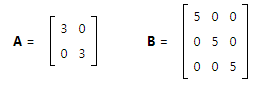• Note that each of these matrices satisfy the defining requirement of a symmetric matrix: A = A' and B = B'.

• Diagonal matrix. A diagonal matrix is a special kind of symmetric matrix. It is a symmetric matrix with zeros in the off-diagonal elements. Two diagonal matrices are shown below.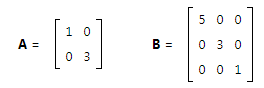• Note that the diagonal of a matrix refers to the elements that run from the upper left corner to the lower right corner.

• Scalar matrix. A scalar matrix is a special kind of diagonal matrix. It is a diagonal matrix with equal-valued elements along the diagonal. Two examples of a scalar matrix appear below.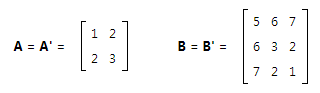These square matrices play a prominent role in the application of matrix algebra to real-world problems. For example, a scalar matrix called the identity matrix is critical to the solution of simultaneous linear equations. (We cover the identity matrix later in the tutorial.)

## Problem 1

Consider the matrices shown below - a, A, B, and C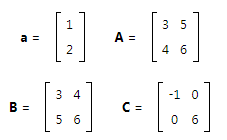Which of the following statements are true?

I.   a is a row matrix
II.  A = B'
III. C is a symmetric matrix

(A) I and II
(B) I and III
(C) II and III
(D) None of the above
(E) All of the above

Solution

The correct answer is (C), as explained below.

• Matrix a is a column vector, not a row matrix
• The transpose of a matrix is created by interchanging corresponding rows and columns. When this is done to matrix B, we see that A = B'.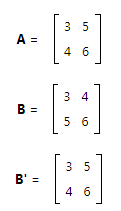• The transpose of C is equal to C; that is C = C'. Therefore, C is a symmetric matrix.
• Note that the off-diagonal elements of matrix C are equal to zero; so matrix C is a diagonal matrix, which is a special kind of symmetric matrix.

The document Types of Matrices - Matrices and Determinants, Business Mathematics & Statistics Notes | Study Business Mathematics and Statistics - B Com is a part of the B Com Course Business Mathematics and Statistics.
All you need of B Com at this link: B Com

## Business Mathematics and Statistics

114 videos|142 docs
 Use Code STAYHOME200 and get INR 200 additional OFF

## Business Mathematics and Statistics

114 videos|142 docs

Track your progress, build streaks, highlight & save important lessons and more!

,

,

,

,

,

,

,

,

,

,

,

,

,

,

,

,

,

,

,

,

,

,

,

,

;